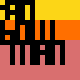# Processing Month, Day 26 - Data Visualisation

Posted by Jan Vantomme on 26 May 2011.
Tags: ,

Today I’ll be mixing two sketches we did this month: the data visualisation sketch we did yesterday and the one about using PDF files for lasercutting. I’ll share the algorithm I used to create these beautiful tangible datasets.The algorithm for drawing the polygon is similar to the one we used yesterday for drawing the bars. We’ll use beginShape() and we need to draw two vertices each time we go through the loop.

``` void renderPolygon(){    float closeX = 0;    float closeY = 0;    beginShape();    for (int i = 0; i < values.length; i++) {        float r = map(values[i], 0, 100, minRadius, maxRadius);        if ( i == 0 ) {            closeX = cos(i * angle) * r;            closeY = sin(i * angle) * r;        }        float x1 = cos(i * angle) * r;        float y1 = sin(i * angle) * r;        float x2 = cos((i + 1) * angle) * r;        float y2 = sin((i + 1) * angle) * r;        vertex(x1, y1);        vertex(x2, y2);    }    endShape(CLOSE);    ellipse( 0, 0, minRadius, minRadius );    ellipse( minRadius/2 + 5, 0, 4, 4 );}  ```

The function for the circles uses the minimum and maximum radius and the lerp() function to calculate the radius for each circle. The distance between each circle is 10%, so the values for each bar will still be readable when the objects are cut.

``` void renderCircles(){    float diff = maxRadius - minRadius;    for (int i = 0; i < 10; i++) {        float r = lerp( minRadius, maxRadius, i / 10.0 ) * 2;        noFill();        ellipse( 0, 0, r, r );    }    ellipse( 0, 0, maxRadius * 2, maxRadius * 2 );}  ```

The output of the sketch looks like this. If you combine this algorithm with the technique I’ve used to output everything to a PDF file, you’ll be able to make beautiful objects made from data.